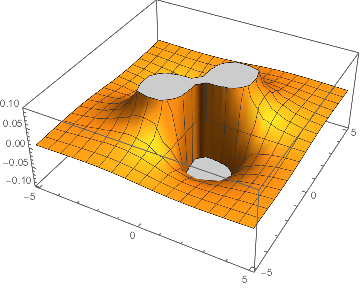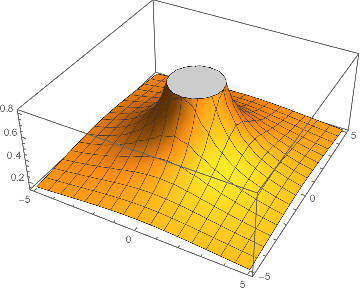## Calculus

Mathematica is useful for many types of mathematical operations, but of particular use are derivatives and integrals.

### Derivatives

Derivatives can be taken using either the function D or the alias ESCpdESC.

For example, to take the first derivative of x^2 one can use either of the following:

 D[x^2,x]

 2 x


or

 Subscript[∂, x]x^2

 2 x


A derivative can be taken of a derivative:

 Subscript[∂, x]Subscript[∂, x]x^2

 2


But this is equivalent to a simple second derivative which Mathematica can also do:

 D[x^2,{x,2}]

 2


or

 Subscript[∂, x, x]x^2

 2


We can also take derivatives in multiple variables:

 Subscript[∂, x, y]1/Sqrt[x^2 + y^2]

 (3 x y)/(x^2 + y^2)^(5/2)


Here's that but formatted better:

$$\frac{3 x y}{\left(x^2+y^2\right)^{5/2}}$$

And then for fun let's plot this:

 dxy=Subscript[∂, x, y]1/Sqrt[x^2 + y^2];
Plot3D[dxy,{x,-5,5},{y,-5,5}]### Integration

Mathematica also knows how to do more integrals than any chemist is likely to need. The function for integration is Integrate although like with derivatives there is an alias via ESCinttESC for indefinite integrals and ESCdinttESC for definite integrals

Here's the standard form for Integrate :

 Integrate[2,x]

 2 x


And here's the alias form:

 ∫2ⅆx

 2 x


And just to show that Mathematica observes the fundamental theorem of calculus like the rest of us:

 ∫∫dxyⅆxⅆy

 1/Sqrt[x^2 + y^2]


Again, here's the same but formatted better:

$$\frac{1}{\sqrt{x^2+y^2}}$$

And we'll plot this too:

 reversedxy=∫∫dxy ⅆxⅆy;
Plot3D[reversedxy,{x,-5,5},{y,-5,5}]### Fun examples:

Mathematica is a great calculus tool and it knows many types of interesting functions so we'll have it integrate the following nasty function for us:

$$P-hash-!!!11573959464626687!!!-hash-4(\cos (\theta ))}{\partial \theta }$$

where $P_i(\cos (\theta ))$ is the $i^{\text{th}}$ Legendre polynomial in $\cos (\theta )$

 Integrate[LegendreP[3,Cos[θ]]Cot[θ]D[LegendreP[4,Cos[θ]],θ],{θ,0,π}]

 0


but Mathematica has absolutely no problem evaluating this integral. It can even tell you that switching the derivative and cot terms makes the integral impossible to evaluate.

 Integrate[LegendreP[3,Cos[θ]]D[Cot[θ]LegendreP[4, Cos[θ]],θ], {θ,0,π}]


$$\int_0^{\pi } \frac{1}{2} \left(-3 \cos (\theta )+5 \cos ^3(\theta )\right) \left(-\frac{1}{8} \left(3-30 \cos ^2(\theta )+35 \cos ^4(\theta )\right) \csc ^2(\theta )+\frac{1}{8} \cot (\theta ) \left(60 \cos (\theta ) \sin (\theta )-140 \cos ^3(\theta ) \sin (\theta )\right)\right) \, d\theta$$

And when it sees that it simply returns the integral unevaluated